How do you... How do you even... This is when you know the problem you are about to be shown, will be annoying. When a friend of mine first introduced this problem, I thought this would be very, VERY simple, to solve. Use some angle properties, use the given similar triangles, and soon enough, a solution will be found. Of course this didn't work. I tried a few other things, same result. Showed it to my family, not much help was gained. They tried what I did. Then it hit me. The goal is to find a specific measure of the triangles. Trigonometry $=$ The Measurement of Triangles. The solution quickly followed using a specific property, and I was like, "Meh. That was quite obvious." Enough ranting, it's time you got a look at the problem itself.

In the diagram below, $\angle ABC = \angle ACB = \angle DEC = \angle CDE$, $\,\overline {BC} = 8$, and $\,\overline {DB} = 2$. Find $\,\overline {AB}$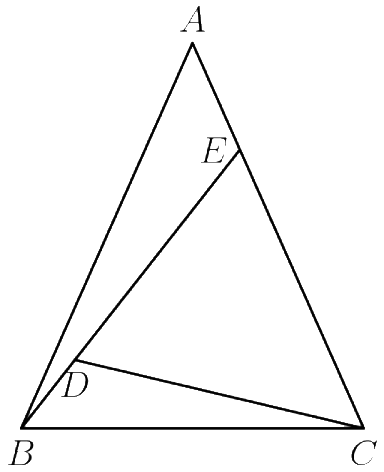When drawing everyting out...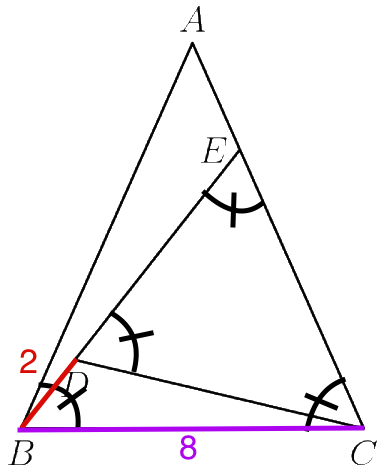Before you continue reading, I highly encourage you attempt this geometry problem. It's an interesting problem, and once you found the concept you need to use to solve it, it's all an easy ride down from there. Following this warning will be the full solution and my thoughts on how I solved this myself. I know I already talked about my thoughts and how I solved this a little in the beginning of this post, but from here on will be everything I thought about.
You have been warned...

The property I thought of (after my 45 minutes of trial-and-error) that we can use to solve for $\,\overline {AB}$ is the The Law of Sines, which states:
$\large \frac{a}{\sin A} = \frac{b}{\sin B} = \frac{c}{\sin C}$

Where $A$ is the angle oposite of $a$, $\,B$ is the angle oposite of $b$, and $C$ is the angle oposite of $c$.

We can rewrite this using the diagram:

$\large \frac{\overline{BC}}{\sin \angle BAC} = \frac{\overline{AC}}{\sin \angle ABC} = \frac{\overline{AB}}{\sin \angle ACB}$

So now that we have this written out, we can start solving for $\,\overline {AB}$. For convenience, I'm going to refer to the angles equivalent to $\,\angle ABC$ as $\,\theta$.

Using the Angle Sum Theorem, $\,\angle BAC = 180 - 2 \theta$. Using this, we can find an expression equal to $\,\overline {AB}$.

$\large \frac{\overline {BC}}{\sin \angle BAC} = \frac{\overline {AB}}{\sin \angle ACB}$

Doing some substitution...

$\large \frac{8}{\sin 2 \theta} = \frac{\overline {AB}}{\sin \theta}$

For where the $\sin 2 \theta$ came from, $\sin 180 - 2 \theta$ when evaluated, is the same as $\sin 2 \theta$. Now for some expansion and evaluation...

$\large \frac{8}{2 \sin \theta \cos \theta} = \frac{\overline {AB}}{\sin \theta}$

$\overline {AB} \sin \theta \cos \theta = 4 \sin \theta$

$\overline {AB} = \large \frac{4}{\cos \theta}$

Now that we have an expression for $\overline {AB}$, we just need to find a value of $\cos \theta$, and that will give us the length of $\overline {AB}$! So now, what can we do? What I first thought (based on the information we were given), if we find two expressions representing the value of the same side length, we can set those two expressions to equal one another, to find a value that makes that equation true. That equation will mostly likely output a value of a function of an angle, as we know very few side lengths, and know no angles (we're hoping it would output a value of $\cos \theta$). Again, this is only what I was thinking when solving this problem at the time. The only reason I thought this, is that I noticed two triangles, that were similar to $\triangle ABC$, contained within $\triangle ABC$.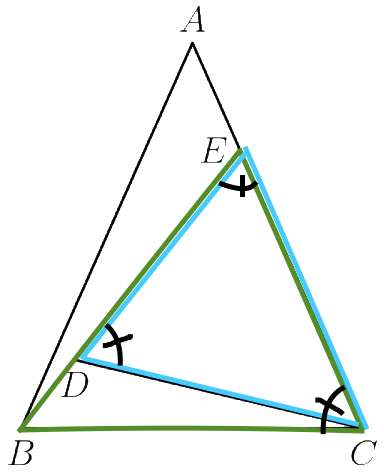We have similar triangles $\triangle DEC$ and $\triangle BEC$. And we know that they are similar as the both triangles share the same angles ($\theta, \theta, and \,180 - \theta$) as the original triangle $\triangle ABC$. And rememeber that side length I mentioned earlier that we could find two expressions for, and use those to solve for its length (that's a mouthful)? That length is $\overline {CE}$! It shares a side length with $\triangle DEC$ and $\triangle BEC$, and we can find our two expressions, by solving for the length of $\overline {CE}$ once, in $\triangle DEC$, and again in $\triangle BEC$. Agian, we're hoping for a value of $\cos \theta$. Starting by solving for $\triangle BEC$...

We are given the length of $\overline {BC} = 8$, which simplifies our job quite a bit. We can do the same thing we did to find an expression for $\overline {AB}$: Use the Law of Sines!

$\large \frac{8}{\sin \theta} = \frac{\overline {CE}}{\sin 2 \theta}$

$\large \frac{8}{\sin \theta} = \frac{\overline {CE}}{2 \sin \theta \cos \theta}$

$\overline {CE} \sin \theta = 16 \sin \theta \cos \theta$

$\overline {CE} = 16 \cos \theta$

We now have a value of $\overline {CE}$ from $\triangle BEC$, time to solve $\overline {CE}$ for $\triangle DEC$...

First off, all though it's not stated, we know the length of $\overline {DE}$. $\triangle BEC$ is an isosceles, where $\angle BEC = \angle ECB$, which also means $\overline {BC} = \overline {BE}$. As $\overline {BC} = 8$, therfore $\overline {BE} = 8$. Since we were told $\overline {DB} = 2$, we can solve $\overline {BE} - \overline {BD} = \overline {DE} = 6$. Now back to the all-mighty, Law of Sines...

$\large \frac{\overline {DE}}{\sin 2 \theta} = \frac{\overline {CE}}{\sin \theta}$

Substitution and expansion...

$\large \frac{3}{\sin \theta \cos \theta} = \frac{\overline {CE}}{\sin \theta}$

$\overline {CE} \sin \theta \cos \theta = 3 \sin \theta$

$\overline {CE} = \large \frac{3}{\cos \theta}$

Great! We're lucky that it came out as a value of $\cos \theta$, but anyways, we have our two expressions, now just to set them equal to one another...

$16 \cos \theta = \large \frac{3}{\cos \theta}$

$16 \cos^2 \theta = 3$

$cos^2 \theta = \large \frac{3}{16}$

$\cos \theta = \large \frac{\sqrt{3}}{4}$

Now that we have our value of $\cos \theta$, we can just substitute this into our original expression for $\overline {AB}$...

$\overline {AB} = \large \frac{4}{\cos \theta}$

$= \large \frac{4}{(\frac{\sqrt{3}}{4})}$

$= \large \frac{16}{\sqrt{3}}$

And there it would be, our solution! Although it might of seemed quite lengthy to get to $\frac{16}{\sqrt{3}}$, it all just revolved around the one concept of the Law of Sines, so not to bad.

Although this is one way to obtain the solution, I'm sure there are other ways to tackle this problem, and I found another way which completely negates our first step, to find an expression for $\overline {AB}$, but adds an extra step to the end.

With our value of $\cos \theta = \frac{\sqrt{3}}{4}$, we can draw a right triangle with this as one of our angles with a bit moving around.

$\theta = \arccos \large \frac {\sqrt{3}}{4}$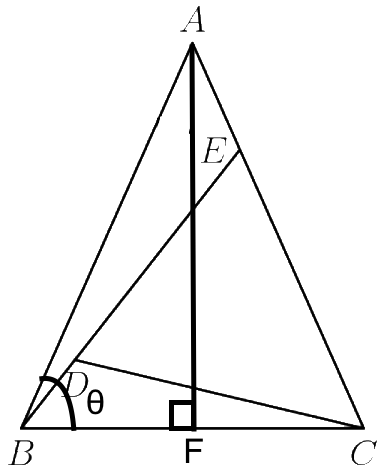We can do this, because as we stated earlier $\theta = any\,angle\,equivalent\,to\, \angle ABC$ (and that's the exact angle we're working with). We also bisected $\overline {BC}$ at $F$ to form the 2 right triangles within our isosceles triangle, so the length of $\overline {BF} = 4$. We can then use some basic trigonometry and evaluation to solve for $\overline {AB}$.

$\theta = \arccos \large \frac {\sqrt{3}}{4}$

$\cos \theta = \large \frac {4}{\overline {AB}}$

$\cos (\arccos \large \frac {\sqrt{3}}{4}) = \large \frac {4}{\overline {AB}}$

$\large \frac {\sqrt{3}}{4} = \large \frac {4}{\overline {AB}}$

${\large \frac {\sqrt{3}}{4}} \overline {AB} = 4$

$\overline {AB} = \large \frac{16}{\sqrt{3}}$

Just another simple way of getting to the exact same answer.

EDIT (JULY 3RD, 2017): RECURSIVE DIVISIONS SOLUTION
This specific solution, is one of my favorites that I have seen. One of my inital attempts was to use the dimensions of the similar triangels and find the common ratio between the side length and the base of the triangle. I knew it could be done, but never put my finger on it. However, when a friend of mine took a look at this problem, after a bit of thought, he managed to come up with this. It's really quite a spectacular of a solution, and this is credited entirely to him (no use of name for privacy reasons). Oh, and I'll be speaking in first person, just so I don't cause any confusion, or make it seem like I'm taking it as mine. Just to be clear.
So the first step is to take the three triangles we know to be similar to one another ($\triangle ABC, \triangle BEC, and \triangle CED$. We know that they are similar due to the fact they all share two common angles, which force them to have a common ratio between the base and a leg of the triangle. This will be important to remember later), and we will $0-index$ them from the original triangle, to the following divisions within one another. I will also now be referring to the triangles by their respective index numbers.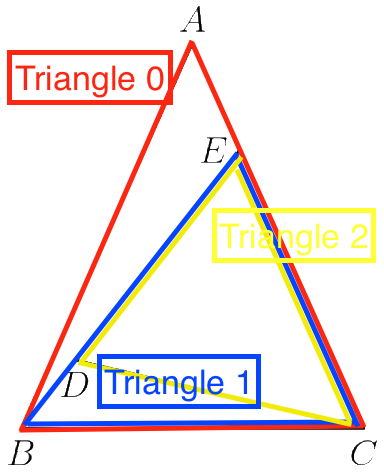Now using the fact that every triangle is similar, and that each progressive triangle was formed by using the base length of the previous triangle to form the leg of the next triangle, we can find a ratio between a dimension (say, the base) of a triangle, and its previous/next triangle, and use that to find the length of $\overline{AB}$. I know that is kind of confusing right now, but trust me, it will makes more sense the more I go on.
So we know the base length of two bases of two triangles ($\triangle 0$, and $\triangle 2$). Since we know that they should share a common ratio, we can right them as a ratio between one another, and hence find said ratio.
$\large \frac{\overline{BC}}{\overline{DE}} = \frac{8}{6}$

$\large = \frac{4}{3}$

So we have a ratio, but the problem with this ratio it's for two divisions. It's for going between $\triangle 0$ and $\triangle 2$. We want one between $\triangle 0$ and $\triangle 1$, or $\triangle 1$ and $\triangle 2$. But this is easy! Since a division in this case is a factor of the previous triangle. This means if we take some dimenstion _a_ of a triangle, multiply it by our ratio once, we will obtain the dimension _a_ of the next division's triangle. For an example, if we have triangle-base $\overline{BC}$, and multiply it by our ratio, we should get the length of triangle-base $\overline{EC}$. Take a look at the diagram if that helps. Essentially, the base length of $\triangle 0$, multiplied by some ratio, we will get the base length of $\triangle 2$, and do that again, we will get the base length of $\triangle 3$. Now if you see, we had to multiply twice to get from $\triangle 0$ to $\triangle 2$. A.K.A., take the square of the ratio. To undo a square, you take the squareroot. So we can undo our two-division ratio, by taking the squareroot of that, to get our one-division ratio.

$\sqrt{\large{\frac{4}{3}}} = \large{\frac{2}{\sqrt{3}}}$

So that's our ratio between a one triangle division. So now we need to find the length of $\overline{AB}$. So we can do what we did originally with the base lengths, only with the legs of the triangle. Larger triangle, over the divided triangle. In this case, $\triangle 0$ over $\triangle 1$.

$\large \frac{\overline{AB}}{\overline{BC}} = \frac{2}{\sqrt{3}}$

$\large \frac{\overline{AB}}{8} = \frac{2}{\sqrt{3}}$

$\overline{AB} = \large \frac{2 \cdot 8}{\sqrt{3}}$

$\overline{AB} = \large \frac{16}{\sqrt{3}}$

And there it is! The answer we had before. I cannot tell you how cool of a solution this is. Simple in concept, but well executed. So major props to my friend for carrying out such a clean solution. I tried this, completely missed the obvious, and he did not. Major props to him.